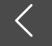# AICPA每日一练之BEC【2016-4-8】

2016-04-08

When calculating a company’s cost of common stock, an analyst evaluates the following four components: risk free rate, stock’s beta coefficient, rate  of return on the market portfolio, and required rate of return on the company’s stock. Which of the following measurement models is being used?

a. Constant growth.

b. Weighted marginal cost of capital.

c. Capital asset pricing.

d. Overall cost of capital.

Choice “c” is correct.

The requirement is to identify the type of valuation model that is being used.

Answer (c) is correct because the capital asset pricing model is calculated using the risk free interest rate, the stock’s beta coefficient, and the estimated return on the market to calculate the cost of equity.

Answers (a), (b), and (d) are incorrect because the factors are used in the capital asset pricing model.

高顿网校温馨提醒

USCPA-美国注册会计师，全球会计*9证，国内人才缺口25万，年薪40W起，如何成为全球财会精英？关注微信号“高顿AICPA”(gaodunaicpa)，让我们一起学习USCPA，加入全球精英财会圈，QQ：2355672450，了解USCPA职业发展， 点击预约USCPA在线说明会
美国注册会计师精彩推荐：

USCPA招生专题
USCPA考试常见问题解析

>>>